﻿ Scalar transport in backstep flow - XSim

# Scalar transport in backstep flow

Update: June 1, 2017
OpenFOAM 4.x

## Case directory

\$FOAM_TUTORIALS/basic/scalarTransportFoam/pitzDaily

## Summary

Using the pre-calculated velocity data, the temperature distribution in a backstep geometry will be solved until 0.1 second later.

The fluid flows in from the inlet region with velocity (10, 0, 0) and out from the outlet region. Set the no-slip condition for the upper and lower surfaces in the Y direction, and the calculation is performed as a two-dimensional problem with a single mesh in the Z direction. The pre-calculated velocity data is placed in the directory "0" and the velocity (U) is not solved during the calculation.

Initially, the temperature of the fluid is set to 0, and a fluid of temperature 1 flows in from the inlet region.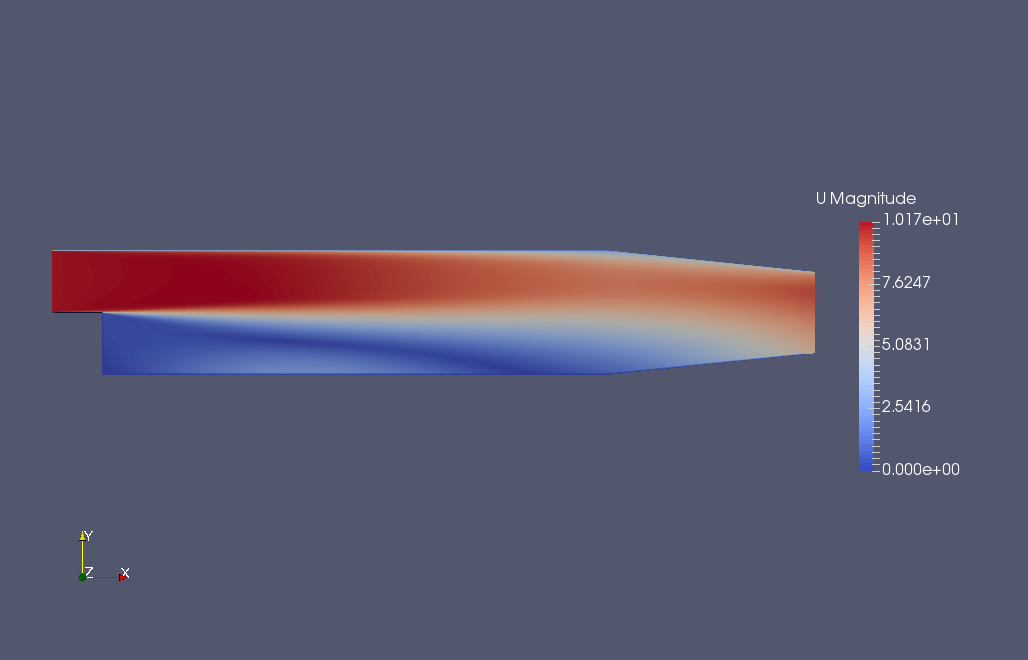The pre-calculated flow velocity

The meshes are as follows.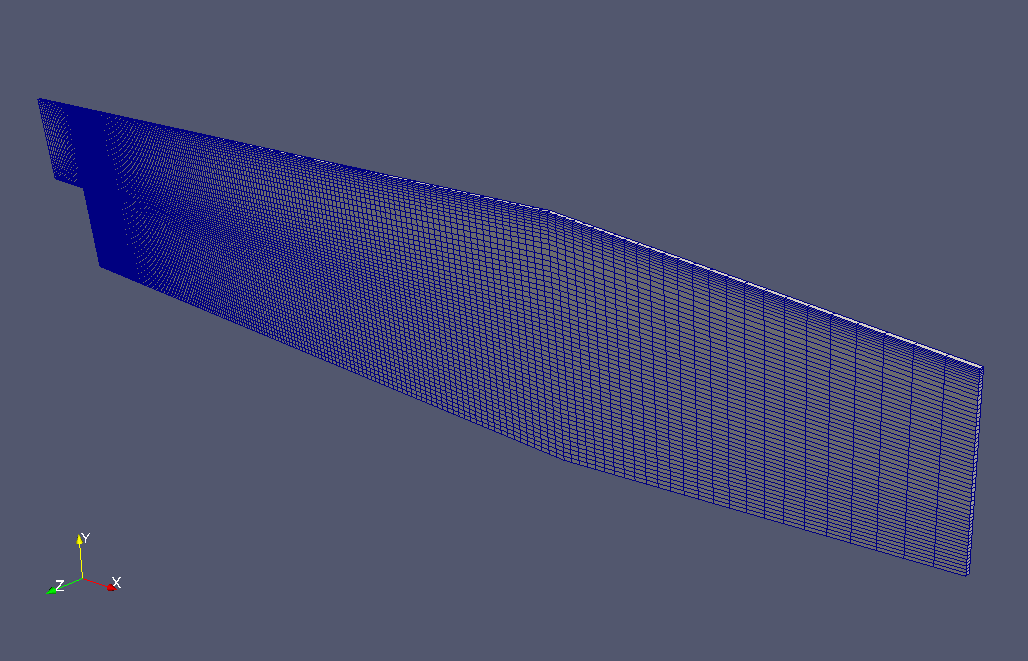Meshes

To visualize the calculated temperature field, check "T" in the "Properties" tab on ParaView.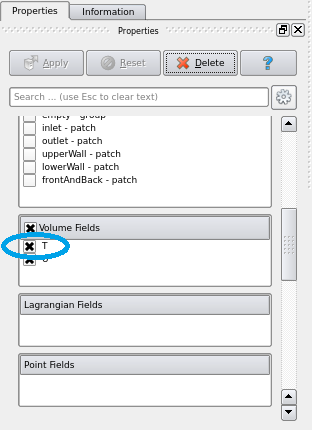Check "T"

The calculation result is as follows.

Temperature distribution (T)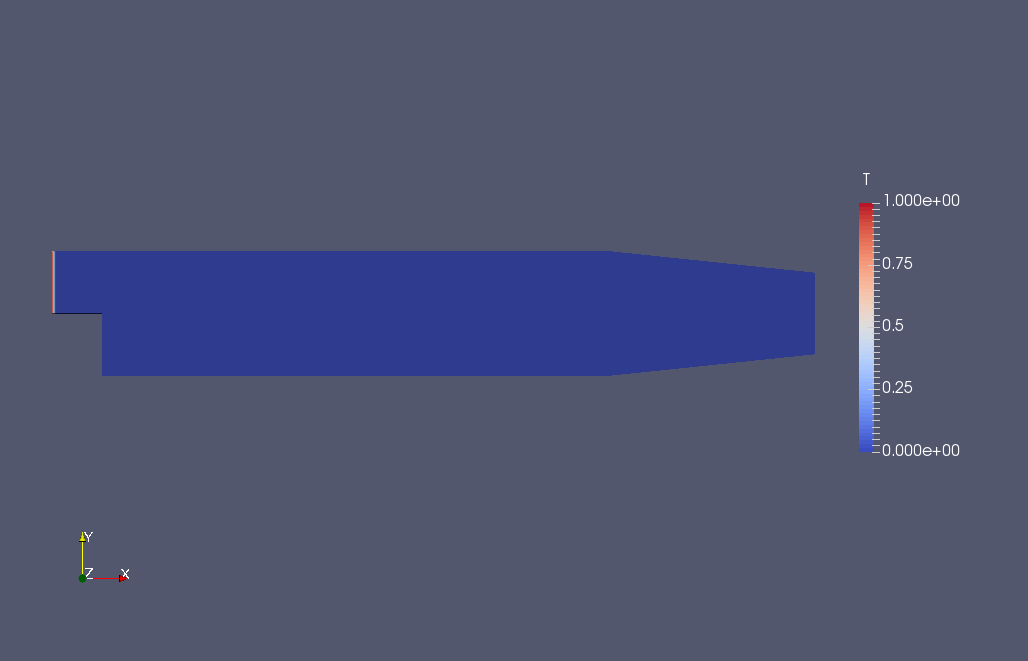Temperature distribution at 0 sec (T)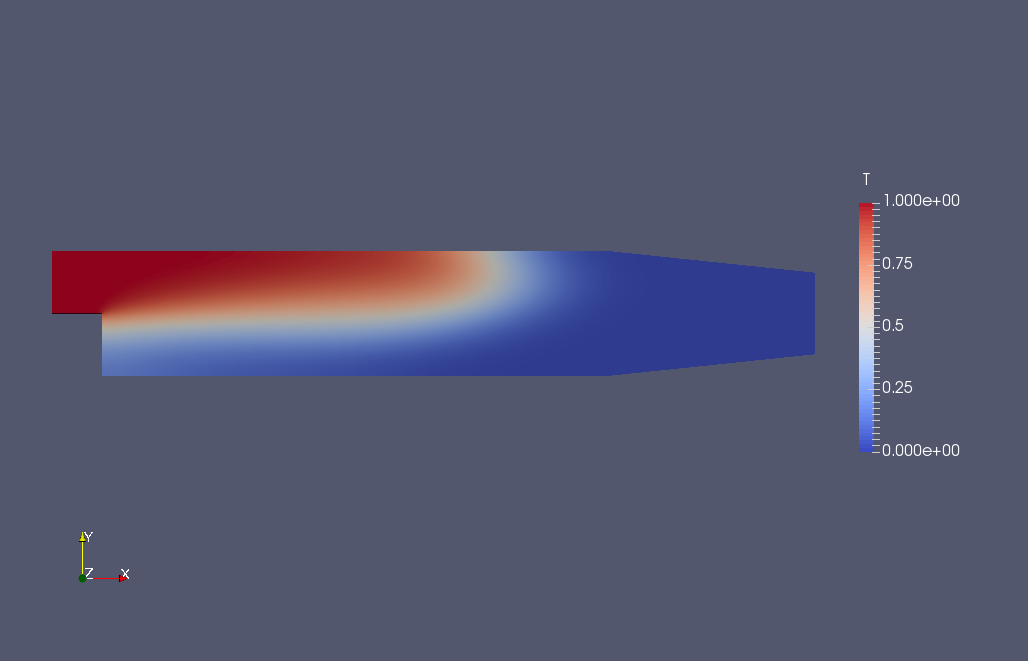Temperature distribution at 0.02 sec (T)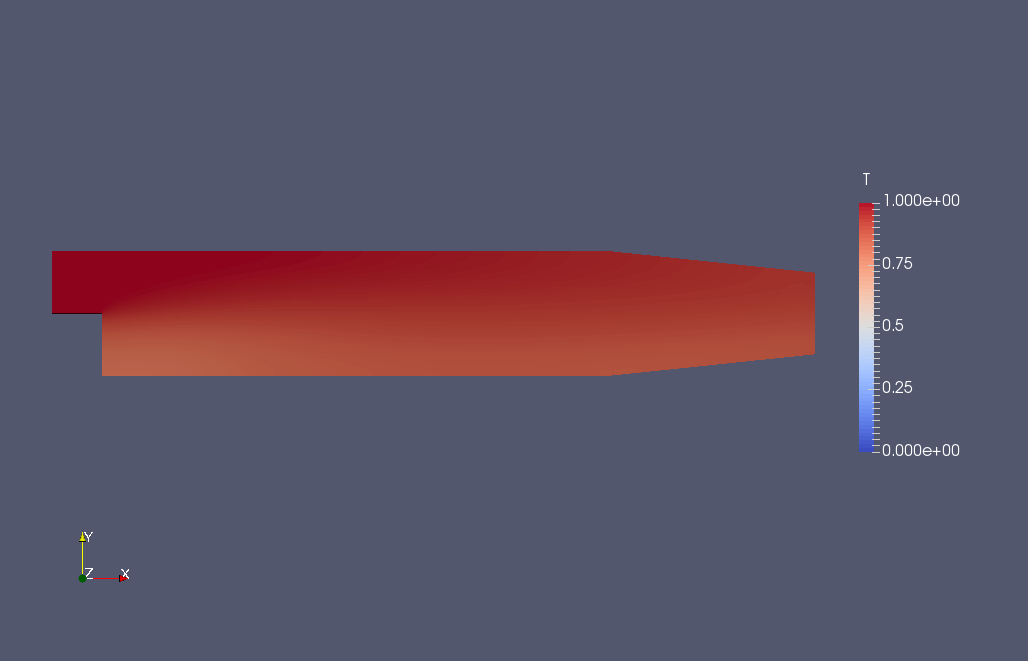Temperature distribution at 0.1 sec (T)

You can see how the temperature is spread by advection and diffusion.

## Commands

cp -r \$FOAM_TUTORIALS/basic/scalarTransportFoam/pitzDaily pitzDaily
cd pitzDaily

blockMesh
scalarTransportFoam

paraFoam

Only temperature wll be solved by the scalarTransportFoam solver.

## Calculation time

--- *Single, Core(TM) i7-2600 CPU @ 3.40GHz 3.40GHz